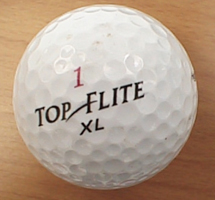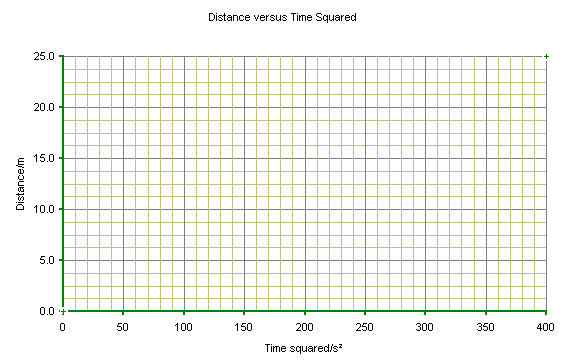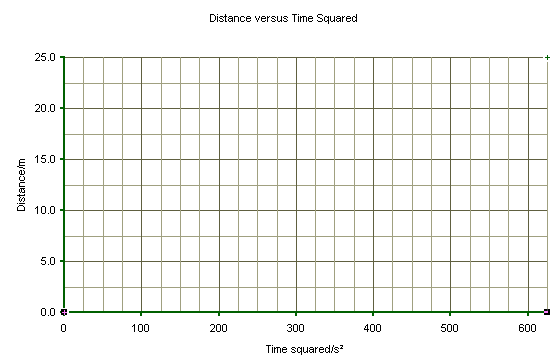### The Golf Ball is Rolling Away!

#### Objectives1. Learn to calculate an acceleration from distance and time data
2. Learn to graph data
3. Learn to draw a best fit line to data
4. Learn to calculate the slope of a best fit line

#### Instructor Discussion Prior to Laboratory

Cover the basics of graphing a best fit line and determining its slope.  Cover the theory of how a linear line can be gotten from quadratic data.

#### Set Up

Measure a total distance of 25 meters along any constantly sloped road or walkway.   Places rocks or other markers every 5 meters.

#### Experimental Procedure

1. Release the golf ball from rest at the start of the downhill course.
2. Time the golf ball as it passes each 5 meter mark.
3. Record your data in the table below.

#### Table

Elapsed Time/s Elapsed Time Squared/s² Distance/m
____________ ____________ 0
____________ ____________ 5
____________ ____________ 10
____________ ____________ 15
____________ ____________ 20
____________ ____________ 25

#### Graphing the Data#### Calculations

1. Fit a best line to the points.
2. Determine the slope.
1. Pick two points, one near each end of the best fit line.
2. Determine the coordinates of the two points, (x1 , y1) and (x2, y2)
3. Use the formula for determining the slope from two points to obtain the slope:
m = (y2- y1) / (x2- x1)
##### slope m = __________________
1. Determine the y-intercept.
1. Use the formula for determining the slope from two points to obtain the slope:
(y - y1) = m(x - x1)
##### y-intercept = _____________
1. Determine the acceleration of the golf ball by multiplying the slope by a factor of two:

#### Questions

1. What will the distance that the golf ball would travel after 40 seconds at the acceleration calculated above?

2. How long would it take the golf ball to go one kilometer if it continued to accelerate at the rate calculated above?